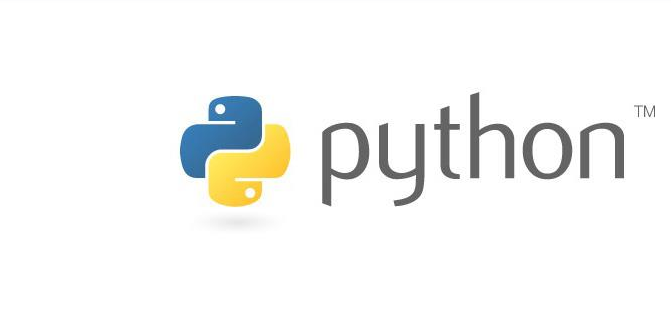• ## 阶乘求和

千次阅读 2017-01-21 13:51:21
求阶乘，阶乘求和，数列求和 * 多种数列求和类型，有 * 1、阶乘fac * 2、阶乘求和facSum * 3、数列求和seriesSum 2/1 ,3/2 ,5/3...... * 4、同数子列sameSum s=a+aa+aaa+....
求阶乘，阶乘求和，数列求和

package arithmetic;

/**
* 多种数列求和类型，有
*      1、阶乘fac
*      2、阶乘求和facSum
*      3、数列求和seriesSum 2/1 ,3/2 ,5/3......
*      4、同数子列sameSum  s=a+aa+aaa+....
*
* @author sunRainAmazing
*
*/
public class SeriesNumberToSum {
public static void main(String[] args) {
int num=5;
System.out.println(num+"的阶乘为："+getFactorialBy_If(num));
System.out.println(num+"的阶乘为："+getFactorialBy_while(num));
//求阶乘的和
System.out.println(num+"的阶乘和为："+getFactorialSum(num));

}

/**
* 求一个非0正整数数自身的阶乘
*      if  else 递归计算
* @param number
* @return
*/
public static long getFactorialBy_If(int number){
long value=1;
if (number == 1){
return value;
}else if (number > 1) {
value = number * getFactorialBy_If(number - 1);
}
return value;
}

/**
* 求一个非0正整数数自身的阶乘
*      while 循环
* @param number
* @return
*/
public static long getFactorialBy_while(int number){
long value=1;
if (number == 1)
return value;
while (number > 1) {
value*=(number--);
}
return value;
}

/**
* 将自身其下的数的阶乘相加
* 如当为5时，5！+4！+3！+2！+1！
* @param number
* @return
*/
public static long  getFactorialSum(int number){
long sum=0;
for(int i=1;i<=number;i++){
sum+=getFactorialBy_while(i);
}
return sum;
}

/**
* 同数子列sameSum  s=a+aa+aaa+....
*
* @param number  要计算的算子
* @param count   要叠加的次数
* @return
*/
public static long getSameSum(int number,int count){
long sum2=0,sum1=0;
for(int i=1;i<count+1;i++){
sum2+=number;
number*=10;
sum1+=sum2;
}
return sum1;
}

/**
* 输入n为偶数时，执行求：     1/2+1/4+...+1/n,
* 当输入n为奇数时，执行求：    1/1+1/3+...+1/n;
* 注意i的步值
*
* @param number 输入的数
* @return
*/
public static double getSeriesSum(int number){
double sum=0;
//计算偶数分子式
if(number%2==0){
for(int i=2;i<=number;i+=2){
sum+=1.0/i;
}
}else{
//计算奇数分子式
for(int i=1;i<=number;i+=2){
sum+=1.0/i;
}
}
return sum;
}

/**
*  求 1/2+3/2+5/3...
* @param number 输入的数
* @return
*/
public static double getSeriesSumUp1(int number){
int x = 2, y = 1;
double sum=0;
for (int i=1; i<number; i++) {
sum +=(x*1.0/y);
x = x + y;//计算分子
y = x - y;//计算分母
}
return sum;
}

/**
*  求 1/2+3/2+5/3...
* @param number 输入的数
* @return
*/
public static double getSeriesSumUp2(int number){
double sum=0;
int temp,i,fenZi=2,fenMu=1;
for (i=1;i<=number;i++){
sum=sum+fenZi*1.0/fenMu; //将各项累加
temp=fenZi;
fenZi=fenZi+fenMu; //将前一项的分子与分母之和作为下一项的分子
fenMu=temp;   //将前一项的分子作为下一项的分母
}
return sum;
}

}

展开全文class
• 使用Python阶乘求和的方法及问题总结发布时间：2020-04-28 09:23:14来源：亿速云阅读：190作者：小新本篇文章和大家了解一下使用Python阶乘求和的方法及问题总结。有一定的参考价值，有需要的朋友可以参考一下，希望...
使用Python阶乘求和的方法及问题总结发布时间：2020-04-28 09:23:14来源：亿速云阅读：190作者：小新本篇文章和大家了解一下使用Python阶乘求和的方法及问题总结。有一定的参考价值，有需要的朋友可以参考一下，希望对大家有所帮助。Python阶乘求和的方法题目描述：获得用户输入的整数n，输出 1!+2!+…+n!的值。如果输入数值为0、负数、非数字或非整数，输出提示信息：输入有误，请输入正整数。方法一：#factTest1def main():a = input()sum = 0if a.isdigit():n = eval(a)if n > 0:fact = 1for i in range(1, n+1):fact *= isum += factprint(sum)else:print("输入有误，请输入正整数")else:print("输入有误，请输入正整数")main()方法二：递归思想#factTest2import syssys.setrecursionlimit(5000)def getSum(i):sum = 0if i==0:return 0else:for x in range(1,i+1):sum += fact(x)return sumdef fact(m):if m==0:return 1else:return m*fact(m-1)def main():n = input()if n.isdigit():a = eval(n)if a>0:result = getSum(a)print(result)else:print("输入有误，请输入正整数")else:print("输入有误，请输入正整数")main()问题总结：当使用递归方法求1024的阶乘时，出现了一个异常：RecursionError: maximum recursion depth exceeded in comparison，超出了递归的最大深度。一些网友提到Python中默认的最大递归深度为1000，但在实际测试中，我的电脑到997的时候就出现异常了，不知道这是由什么决定的。因此，为了能够计算1024的阶乘，需要为最大递归深度赋予一个更大的值。这里可以采用以下方法：import syssys.setrecursionlimit(5000) #修改为5000另外，也可以查看最大递归深度：import syssys.getrecursionlimit()  # output:1000关于使用Python阶乘求和的方法及问题总结就分享到这里了，当然并不止以上和大家分析的办法，不过小编可以保证其准确性是绝对没问题的。希望以上内容可以对大家有一定的参考价值，可以学以致用。如果喜欢本篇文章，不妨把它分享出去让更多的人看到。
展开全文• Python阶乘求和的方法题目描述：获得用户输入的整数n，输出 1! 2! … n!的值。如果输入数值为0、负数、非数字或非整数，输出提示信息：输入有误，请输入正整数。(推荐学习：Python视频教程)方法一：#factTest1def ...Python阶乘求和的方法题目描述：获得用户输入的整数n，输出 1! 2! … n!的值。如果输入数值为0、负数、非数字或非整数，输出提示信息：输入有误，请输入正整数。(推荐学习：Python视频教程)方法一：#factTest1def main():a = input()sum = 0if a.isdigit():n = eval(a)if n > 0:fact = 1for i in range(1, n 1):fact *= isum  = factprint(sum)else:print("输入有误，请输入正整数")else:print("输入有误，请输入正整数")main()方法二：递归思想#factTest2import syssys.setrecursionlimit(5000)def getSum(i):sum = 0if i==0:return 0else:for x in range(1,i 1):sum  = fact(x)return sumdef fact(m):if m==0:return 1else:return m*fact(m-1)def main():n = input()if n.isdigit():a = eval(n)if a>0:result = getSum(a)print(result)else:print("输入有误，请输入正整数")else:print("输入有误，请输入正整数")main()问题总结：当使用递归方法求1024的阶乘时，出现了一个异常：RecursionError: maximum recursion depth exceeded in comparison，超出了递归的最大深度。一些网友提到Python中默认的最大递归深度为1000，但在实际测试中，我的电脑到997的时候就出现异常了，不知道这是由什么决定的。因此，为了能够计算1024的阶乘，需要为最大递归深度赋予一个更大的值。这里可以采用以下方法：import syssys.setrecursionlimit(5000) #修改为5000另外，也可以查看最大递归深度：import syssys.getrecursionlimit()  # output:1000
展开全文• Python阶乘求和的方法题目描述：获得用户输入的整数n，输出 1!+2!+…+n!的值。如果输入数值为0、负数、非数字或非整数，输出提示信息：输入有误，请输入正整数。方法一：#factTest1def main():a = input()sum = 0if ...
Python阶乘求和的方法题目描述：获得用户输入的整数n，输出 1!+2!+…+n!的值。如果输入数值为0、负数、非数字或非整数，输出提示信息：输入有误，请输入正整数。方法一：#factTest1def main():a = input()sum = 0if a.isdigit():n = eval(a)if n > 0:fact = 1for i in range(1, n+1):fact *= isum += factprint(sum)else:print("输入有误，请输入正整数")else:print("输入有误，请输入正整数")main()方法二：递归思想#factTest2import syssys.setrecursionlimit(5000)def getSum(i):sum = 0if i==0:return 0else:for x in range(1,i+1):sum += fact(x)return sumdef fact(m):if m==0:return 1else:return m*fact(m-1)def main():n = input()if n.isdigit():a = eval(n)if a>0:result = getSum(a)print(result)else:print("输入有误，请输入正整数")else:print("输入有误，请输入正整数")main()问题总结：当使用递归方法求1024的阶乘时，出现了一个异常：RecursionError: maximum recursion depth exceeded in comparison，超出了递归的最大深度。一些网友提到Python中默认的最大递归深度为1000，但在实际测试中，我的电脑到997的时候就出现异常了，不知道这是由什么决定的。因此，为了能够计算1024的阶乘，需要为最大递归深度赋予一个更大的值。这里可以采用以下方法：import syssys.setrecursionlimit(5000) #修改为5000另外，也可以查看最大递归深度：import syssys.getrecursionlimit() # output:1000以上就是Python阶乘求和的方法的详细内容，感谢大家的学习和对的支持。
展开全文• Python阶乘求和的方法题目描述：获得用户输入的整数n，输出 1!+2!+…+n!的值。如果输入数值为0、负数、非数字或非整数，输出提示信息：输入有误，请输入正整数。(推荐学习：Python视频教程)方法一：#factTest1def ...
• 在本篇文章里小编给大家整理的是关于Python阶乘求和的代码实例，有需要的朋友们可以跟着学习下。
• 需求：阶乘：也是数学里的一种术语；阶乘指从1乘以2乘以3乘以4一直乘到所要求的数；在表达阶乘时，就使用“！”来表示。如h阶乘，就表示为h!；阶乘一般很难计算，因为积都很大。提问：求1+2!+3!+...+20!的和实现环境...
• 需求：阶乘：也是数学里的一种术语；阶乘指从1乘以2乘以3乘以4一直乘到所要求的数；在表达阶乘时，就使用“！”来表示。如h阶乘，就表示为h!；阶乘一般很难计算，因为积都很大。提问：求1+2!+3!+...+20!的和实现环境...
• 需求：阶乘：也是数学里的一种术语；阶乘指从1乘以2乘以3乘以4一直乘到所要求的数；在表达阶乘时，就使用“！”来表示。如h阶乘，就表示为h!；阶乘一般很难计算，因为积都很大。提问：求1+2!+3!+...+20!的和实现环境......